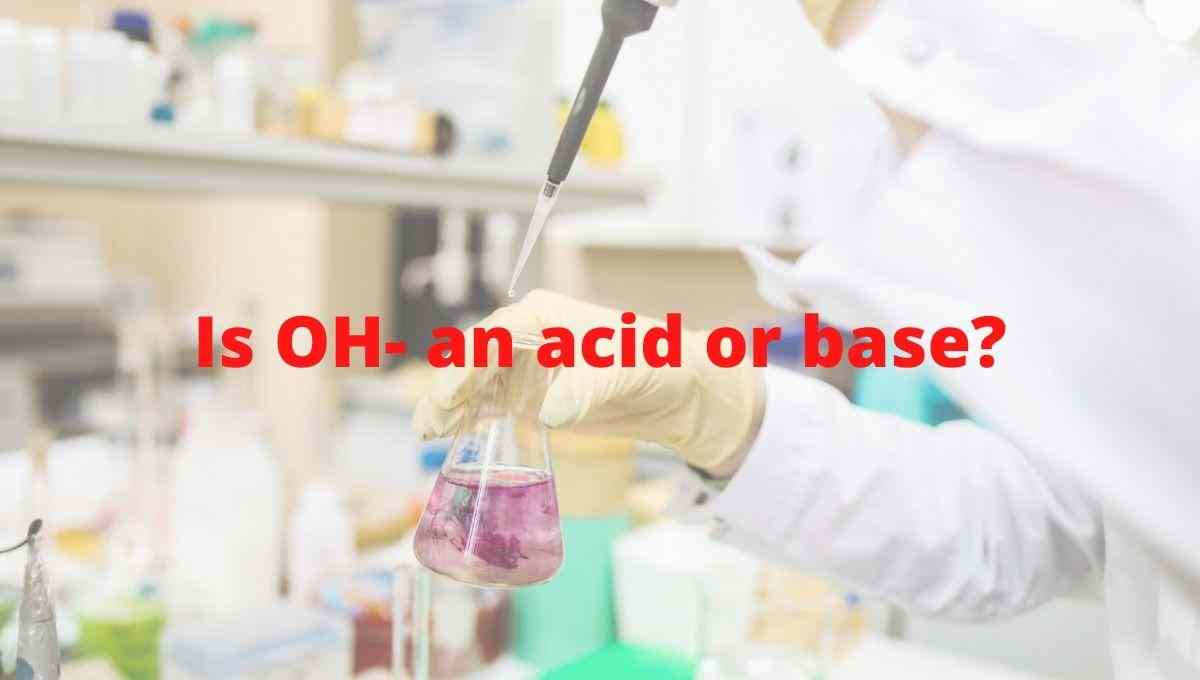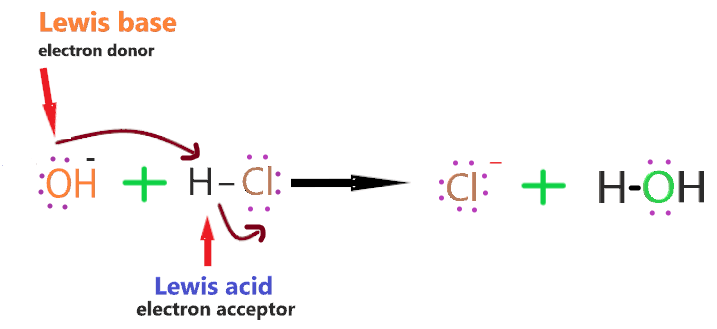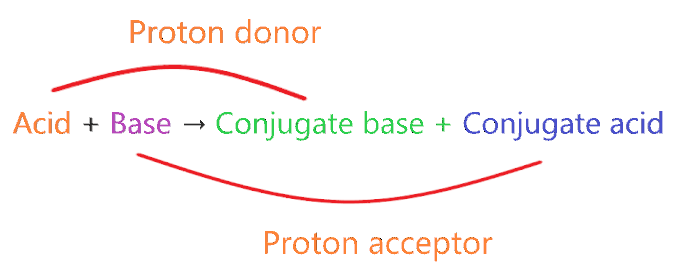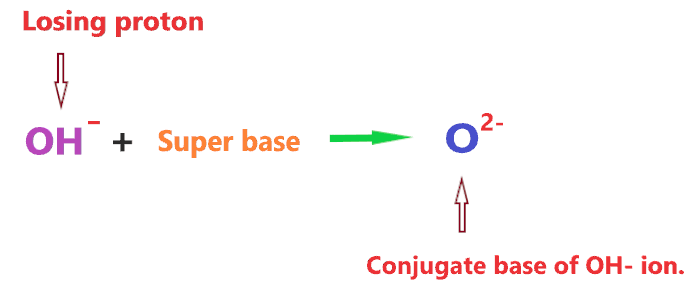# Is Hydroxide ion (OH-) an acid or base? - Conjugate base or Conjugate acid

Home  > Chemistry Article > Is OH- an acid or base?Hydroxide ion carries a negative charge and is made up of one hydrogen and one oxygen joined together by a single covalent bond. It has a chemical formula OH. It is a diatomic ion also called hydroxyl or hydroxyl radical. It is used to produce fuel cells.

In this article, we will discuss Is OH an acid or base? It’s conjugate acid or base pairs, etc?

So, Is OH an acid or base? OH is a base because in an aqueous solution it can accept a proton from an acid to form a water molecule(H2O) and anything that accept the H+ ions in an aqueous solution is known as a base in chemistry.

 Name of Molecule Hydroxide ion Chemical formula OH– Molar mass 35.04 g·mol−1 Nature Base Conjugate acid H2O Conjugate base O2-
Page Contents

## Why OH- is a base?

To Know Why OH is acting as a Base? We have to look into the famous theory given by Arrhenius for the base compounds.

According to Arrhenius theory, the compound is said to be Arrhenius base when it produces OH ion through ionization or through dissociation in water and increases the concentration of OH ions in an aqueous solution.So, we can say OH is an Arrhenius base according to its definition.

There is some good example of Arrhenius base that contains the unit of OH in their chemical formula such as NaOH, KOH, LiOH, Ba(OH)2, Ca(OH)2, etc.

All these, when dissolved in an aqueous solution produce OH ions, hence, increase the concentration of OH in the solution which further raises the pH of a solution.

Now we look for another most important acid-base theory that is the Bronsted-Lowry theory.

This theory states a compound is classified as a base when it accepts the proton from another compound.

• A Bronsted-Lowry acid is a proton (hydrogen ion) donor.
• A Bronsted-Lowry base is a proton (hydrogen ion) acceptor.

Let’s check whether OH ion fulfills the requirement for classifying as Bronsted-Lowry base or not.

Consider the reaction of OH with H2CO3H2CO3 is a Bronsted-Lowry acid because it has a proton to donate, hence, when reacting with OH ion, it donates one proton, this proton can be accepted by the OH ion and forms a water molecule(H2O).

So, we can say, OH ion is a Bronsted-Lowry base as it accepts the proton when reacts with Bronsted-Lowry acids such as H2CO3 or anything that releases H+ ions.

## Is OH- Lewis base or acid?

Lewis’s theory is a very important acid-base theory to check whether a compound (OH) acid or base?

According to the Lewis theory, a compound is said to be acid when it accepts the pair of electrons and a compound is said to be base when it donates the pair of electrons.

⇒ Lewis acid → lone pair acceptor

⇒ Lewis base → lone pair donator

Is OH Lewis acid or base? To know this first we should know what type of compound is Lewis acid and Lewis base.

Lewis acid: They are electron-loving compounds and are also known as electrophilic compounds that accept the electron pair from Lewis bases.

Some examples of Lewis acids that can accept the pair of electrons from another species.

1. The molecules or ions with an incomplete octet of electrons. Example – BF3, BCl3, AlF3, etc.
2. The molecule that central atom has an empty d-orbital. Example- SiCl4 (1s22s22p63s23p3d0).
3. The compounds that the central atom formed multiple bonds with the adjacent atoms. Example – SiO2 (O = Si = O), CO2, SO2, etc.
4. Simple cations like H+, Na+, Mg+2, Al+3, etc. are lewis acids since they are able to accept the electrons. ( Exception NH4+, PH4+ are not a lewis acid)

Lewis base: They are electron-rich species and also known as nucleophile compounds that donate the electron pairs to the Lewis acids.

Some examples of Lewis bases that can donate the pair of electrons to other species.

1. The negative ion such as H, OH, F, etc.
2. Species that have lone pair of electrons. Example – NH3, H2O, CH3, etc.

The Hydroxide ion (OH) ion acts as lewis’s base because it has three lone pairs of electrons and it can easily donate these pairs of electrons to form a covalent bond with another compound acceptor.Take an example to understand How does OH acts as Lewis base when reacts with Lewis acid such as HCl.The electrons in the hydrogen-chlorine bonds attracted towards the chloride ion because of it high electronegativity leaving hydrogen slightly positive and chlroine negative.

The lone pair on the oxygen atom of OH ion attracted towards hydrogen atom in HCl molecule.

As it approaches it, the electrons in the hydrogen-chlorine bond are repelled still further towards the chlorine.

Eventually, a co-ordinate bond is formed between the hydroxide ion and the hydrogen, and the chlorine breaks away as a chloride ion.

The whole HCl molecule acts as Lewis acid as it accept the lone pair from hydroxide ion, and in this process it breaks up. [Concept of lewis acid & base)

So, HCl accepts the lone pair of the electron, therefore, it is Lewis acidand OH ion donates the lone pair of the electron, therefore, it is Lewis base.

## What is Conjugate acid and base of OH- ion?

In technical terms, Compounds differentiated from each other by a single proton(H+) are said to be Conjugate acid-base pairs.

In simple terms, when the proton is removed from parent acid then the compound is formed which is called the conjugate base of that acid and when the proton is added to the parent base then the compound is formed which is called conjugate acid of that base.

Concept of Conjugate acid-base pair-• A very weak acid forms the strong conjugate base.
• A very weak base forms strong conjugate acid.
• A very strong acid forms the weak conjugate base.
• A very strong base forms weak conjugate acid.

⇒ Very weak means it doesn’t act as acid or base when dissolved in an aqueous solution.

⇒ Very strong means, acid or base ionizes 100% when dissolved in an aqueous solution.

Example 1: NH3 + H+ → NH4+

Here in this reaction, NH3 is a weak base and we know a weak base always forms a conjugate acid(not necessarily the strong one).

So, NH4is the conjugate acid of NH3.

Example 2: HCl → H+ Cl

In this reaction, HCl is a very strong acid and we know very strong acid always forms the weak conjugate base by donating one proton.

So, Cl is the weak conjugate base of HCl.

Example 3: CH3COOH + H2O → CH3COO + H3O+

Here in this reaction, CH3COOH is a weak acid that donates the proton to form CH3COO, which means CH3COO– is the conjugate base of CH3COOH.

H2O behaves as the base because it accepts the proton from CH3COOH to form H3O+, which means H3O+ is the conjugate acid of H2O.

So, What is the conjugate acid of OH ion? Water(H2O) is the conjugate acid of OH ion as conjugate acid is formed when H+ is added to the given species.

So, OH is a base and when H+ is added to it, it forms an H2O molecule.Now, What is the conjugate base of OH ion? Oxide anion(O2-) is the conjugate base of OH ion as the conjugate base is formed when H+ is removed from the given species.

So, given species is OH ion, when H+ is removed from it, we get O2-.

However, it should be noted that deprotonation or removal of a proton from OH ion cannot be done in an aqueous solution at ordinary pH. OH ion can only lose  H+ ion in front of the super base.

Superbases are stronger than hydroxide ions and cannot be kept in water.

Hence, when OH ion reacts with a super base, then it will lose a proton and forms an oxide anion(O2-).## Uses of Hydroxide ion

1. It is used to produce disinfectants.
2. It is used as a catalyst in some reactions.
3. It is used in the extraction of alumina.
4. It is also used as a nucleophilic reagent.
5. It is used to produce hydrogen cells.

## Summary

• Is Hydroxide ion (OH) an acid or base? OH is a base because of its “proton accepting” ability in aqueous solution, also, when OH ions are dissolved in solution, the pH value of the solution gradually rises which shows its basic nature.
• The conjugate acid of OH is H2O as conjugate acid is formed when H+ is added to the given species.
• The conjugate base of OH is O2- as the conjugate base is formed when H+ is removed from the given species.
• OH ion is acting as an Arrhenius base and Bronsted-Lowry base.
• OH ion also acts as a Lewis base because it has three unpaired electrons and is able to donate the pair of electrons to Lewis acids for covalent bond formation.
##### Subscribe to Blog via Email

Join 2 other subscribers

Share it...